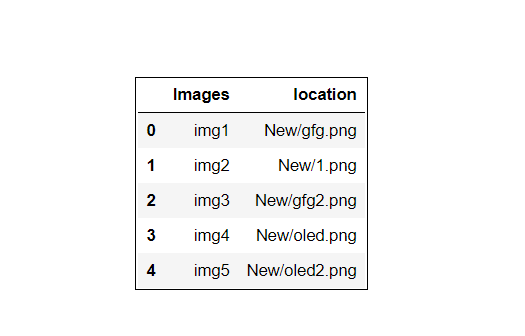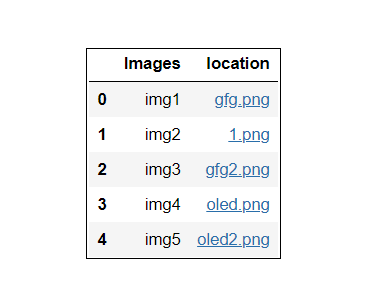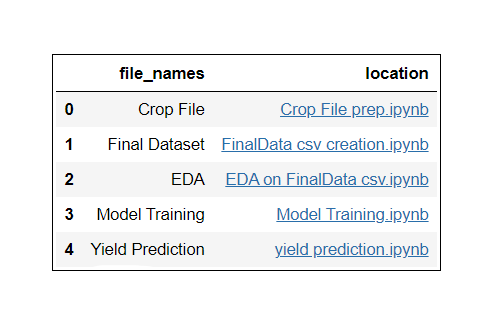Open in App
Not now

# How to create a table with clickable hyperlink to a local file in Pandas?

• Last Updated : 15 Mar, 2021

If you are familiar with Data Science or Machine Learning field then we can definitely say that we are going to learn something new from this article, So let’s begin. Here in this article, we will learn how to create a clickable hyperlink of the local file path using pandas. It is not a very complicated task, we can easily do it in only 4 to 5 steps. Let’s dig deeper into it and talk about required libraries.

• Pandas: It is one of the most favorite and popular libraries in python for data wrangling and analysis. Pandas is the backbone of all Data Science and Machine Learning projects. It is one of the most important tools for Data Scientists and Analysts. Some features of pandas are: Data Handling, Alignment and indexing, Handling missing data, Cleaning data, Merging and joining of datasets, and much more. Here as well we will use pandas to create a dataset.
• OS library: The OS module present in python also plays a very important role in various projects. It provides lots of functions for interacting with the operating system. It includes many functions to interact with the file system as well. Here we have to work with local files that’s why we need an os module to handle them.

Steps Needed

Step 1: Import required libraries.

## Python3

 `# Importing required libraries``import` `pandas as pd``import` `os as o`

Step 2: Creating a dataset using a dictionary and list.

## Python3

 `# Creating dataset of location of files.``dataset ``=` `[``dict``(Images ``=` `'img1'``, location ``=` `r``'New/gfg.png'``),``           ``dict``(Images ``=` `'img2'``, location ``=` `r``'New/1.png'``),``           ``dict``(Images ``=` `'img3'``, location ``=` `r``'New/gfg2.png'``),``           ``dict``(Images ``=` `'img4'``, location ``=` `r``'New/oled.png'``),``           ``dict``(Images ``=` `'img5'``, location ``=` `r``'New/oled2.png'``)]`

Here in the above code snippet, we are creating a small dataset of the local file’s paths present in our local system. First, we are creating a dictionary where the image name acts as a unique key and the path of an image is acts as the value of a unique key here. Finally, converting the dictionary into a list so further we can easily convert the list into pandas dataframe.

Step 3: Converting list into Dataframe and printing it.

## Python3

 `# Converting list into pandas dataframe ``df ``=` `pd.DataFrame(dataset)`` ` `# printing the dataframe``df`

Output :Till Step 2 our dataframe was in list form so in Step 3 we are converting it into pandas dataframe so we can easily apply dozens of operations on it for better analysis. Currently, the path of images is not in clickable form, we have to perform some tricky operation on it to convert in into clickable hyperlink form.

Step 4: Creating a function to convert the path into Clickable form.

## Python3

 `# Function to convert file path into clickable form.``def` `fun(path):``   ` `    ``# returns the final component of a url``    ``f_url ``=` `o.path.basename(path)``     ` `    ``# convert the url into link``    ``return` `'{}'``.``format``(path, f_url)`

In the above code snippet, we have created a function that will convert the file path(location of file) into a clickable hyperlink form.

Step 5: Applying the function on column “location” which will convert path into clickable hyperlink form. Now, whenever we will print the dataframe we will get our required to be output.

## Python3

 `# applying function "fun" on column "location".``df ``=` `df.style.``format``({``'location'` `: fun})`

Output:Finally, we have successfully converted our local file path into a clickable hyperlink form. Let’s check out some examples.

Example 1: Creating a Dataframe that contains the clickable hyperlink path of images present in our local system.

## Python3

 `# Step 1 : Importing required modules``import` `pandas as pd``import` `os`` ` `# Step 2 : Creating dataset of local path images``dataset ``=` `[``dict``(Images``=``'img1'``, location``=``r``'New/gfg.png'``),``           ``dict``(Images``=``'img2'``, location``=``r``'New/1.png'``),``           ``dict``(Images``=``'img3'``, location``=``r``'New/gfg2.png'``),``           ``dict``(Images``=``'img4'``, location``=``r``'New/oled.png'``),``           ``dict``(Images``=``'img5'``, location``=``r``'New/oled2.png'``)]`` ` `# Step 3 : Converting list into dataframe``df ``=` `pd.DataFrame(dataset)`` ` `# Function to convert file path into clickable form.`` ` ` ` `def` `fun(path):``   ` `    ``# returns the final component of a path``    ``f_url ``=` `os.path.basename(path)``     ` `    ``# convert the path into clickable link``    ``return` `'{}'``.``format``(path, f_url)`` ` ` ` `# applying function "fun" on column "location".``df ``=` `df.style.``format``({``'location'``: fun})`` ` `# Step 5 : Finally printing Dataframe``df`

Output :Example 2: Creating a Dataframe containing a clickable hyperlink path of files present in our local system.

## Python3

 `# Step 1 : Importing required modules``import` `pandas as pd``import` `os`` ` `# Step 2 : Creating dataset of files``dataset ``=` `[``dict``(``  ``file_names``=``'Crop File'``, location``=``r``'ML/Crop File prep.ipynb'``),``            ` `           ``dict``(``  ``file_names``=``'Final Dataset'``, location``=``r``'ML/FinalData csv creation.ipynb'``),``            ` `           ``dict``(``  ``file_names``=``'EDA'``, location``=``r``'ML/EDA on FinalData csv.ipynb'``),``            ` `           ``dict``(``  ``file_names``=``'Model Training'``, location``=``r``'ML/Model Training.ipynb'``),``            ` `           ``dict``(``  ``file_names``=``'Yield Prediction'``, location``=``r``'ML/yield prediction.ipynb'``)]`` ` `# Step 3 : Converting list into dataframe``df ``=` `pd.DataFrame(data)`` ` `# Function to convert file path into ``# clickable hyperlink form.``def` `fun(path):``   ` `    ``# returns the final component of a path``    ``f_url ``=` `os.path.basename(path)``     ` `    ``# convert the path into clickable hyperlink``    ``return` `'{}'``.``format``(path, f_url)`` ` ` ` `# Step 4 : applying make_clikable function ``# on column path.``df ``=` `df.style.``format``({``'location'``: fun})`` ` `# Step 5 : Finally printing Dataframe``df`

Output:Note: Whenever we are trying to load local files from the browser we are continuously getting an error ‘Not allowed to load local resources’ in our browser. If we Google this problem and came to know that it is happening due to some security issue of our system. But there is a way we can solve this problem without disturbing our system security, put the required files in the same working directory in which we are working. In the above examples, we created a folder and put all required files in it, and finally easily load them without any issue. In Example 1 we created a folder named “New” in my working directory and put all the required files in it similarly in Example 2 as well.

My Personal Notes arrow_drop_up